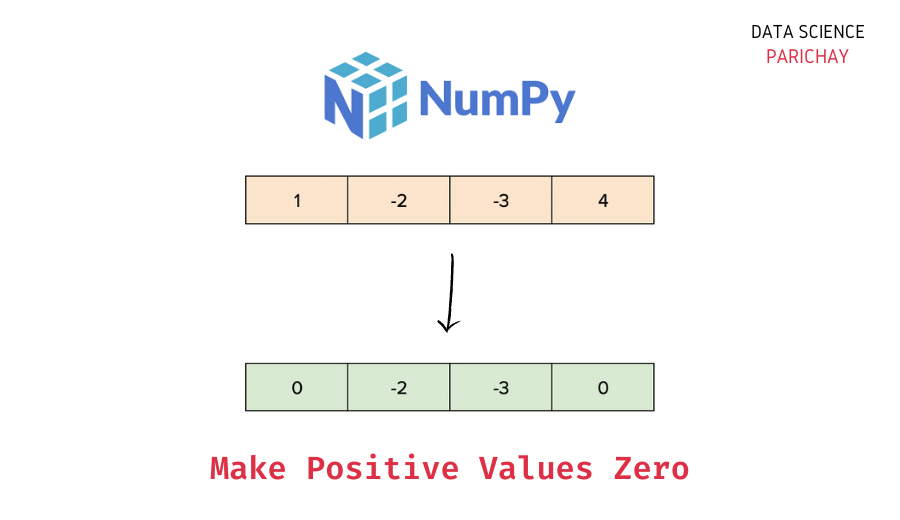# Numpy – Make All Positive Values Zero in Array

The Numpy library in Python comes with a number of useful functions and methods to work with and manipulate the data in arrays. In this tutorial, we will look at how to make all the positive values zero in a Numpy array with the help of some examples.

## Steps to replace positive values with zero in Numpy

You can use boolean indexing to make all the positive values in a Numpy array zero. The following is the syntax –

```# set positve values to zero
ar[ar > 0] = 0```

It replaces the positive values in the array `ar` with `0`.

Let’s now look at a step-by-step example of using this syntax –

### Step 1 – Create a Numpy array

First, we will create a Numpy array that we will use throughout this tutorial.

```import numpy as np

# create a numpy array
ar = np.array([-2, -1, 0, 1, 2, 3, -4])
# display the array
print(ar)```

Output:

`[-2 -1  0  1  2  3 -4]`

Here, we used the `numpy.array()` function to create a one-dimensional Numpy array containing some numbers. You can see that the array has both positive and negative values (along with a zero).

### Step 2 – Set positive values to zero using boolean indexing

Using boolean indexing identify the values that are greater than zero and then set them to zero using the equality operator (`=`).

📚 Data Science Programs By Skill Level

Introductory

Intermediate ⭐⭐⭐

🔎 Find Data Science Programs 👨‍💻 111,889 already enrolled

Disclaimer: Data Science Parichay is reader supported. When you purchase a course through a link on this site, we may earn a small commission at no additional cost to you. Earned commissions help support this website and its team of writers.

First, we will specify our boolean expression `ar > 0` and then set the array values satisfying this condition to zero.

For example, let’s replace all the positive values in the above array with zeros.

```# set positive values to zero
ar[ar > 0] = 0
# display the array
print(ar)```

Output:

`[-2 -1  0  0  0  0 -4]`

We replace all the positive values in the above array with zeros.

To understand what’s happening here, let’s look under the hood. Let’s see what we get from the expression `ar > 0`

```# create a numpy array
ar = np.array([-2, -1, 0, 1, 2, 3, -4])
# result of boolean expression ar > 0
ar > 0```

Output:

`array([False, False, False,  True,  True,  True, False])`

We get a boolean array. The boolean values in this array represent whether a value at a particular index satisfies the given condition or not (in our case whether the element is greater than 0 or not).

When we do `ar[ar > 0] = 0`, we are essentially setting the values in the array where the condition evaluates to `True` to 0.

You can similarly filter a Numpy array for other conditions as well.

## Summary – Make positive values zero in Numpy

In this tutorial, we looked at how to replace all the positive values in a Numpy array with zeros. The following is a short summary of the steps mentioned –

1. Create a Numpy array (skip this step if you already have an array to operate on).
2. Use boolean indexing to find the positive values and then set them to zero – `ar[ar > 0] = 0`

You might also be interested in –

•